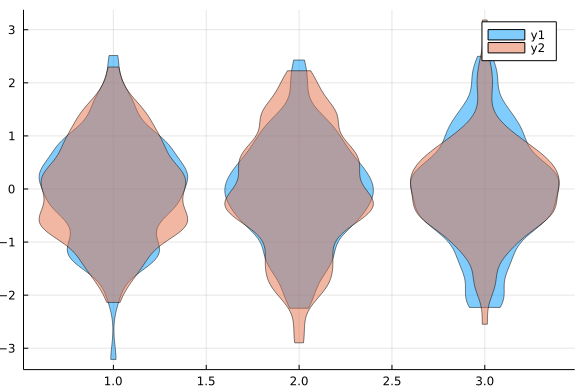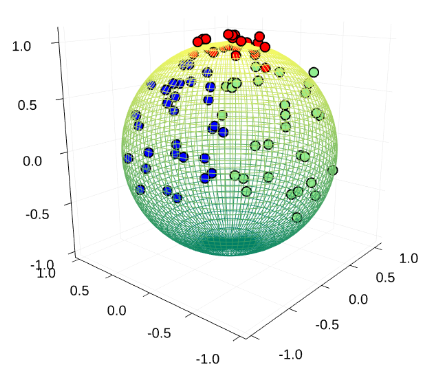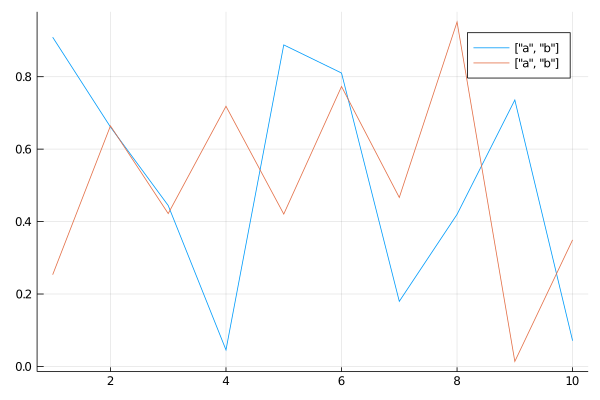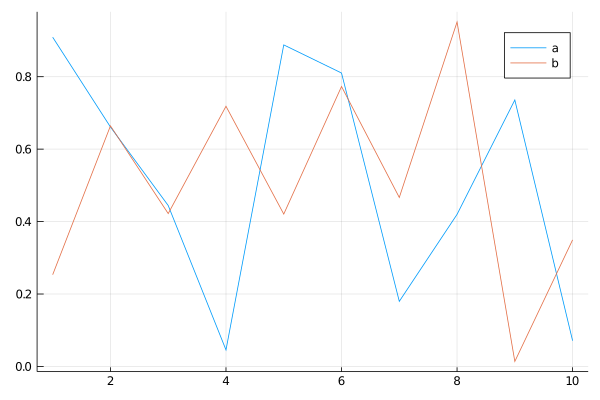# Plot¶

## Plot from server¶

local $ssh -X rocky rocky$ tmux ... # open a tmux session if you like

## Violin Plot¶

using StatsPlots
violin(repeat([1,2,3],outer=100),randn(300), alpha = 0.5)
violin!(repeat([1,2,3],outer=100),randn(300), alpha = 0.5)applications: proj_offset

## 等高线图 (contour)¶

using Plots
using Distributions

x_grid = range(-2, 2, length=100)
y_grid = range(-2, 2, length=100)
Sigma = [1 0.9; 0.9 1];
contour(x_grid, y_grid, (x, y)->pdf(MvNormal([0, 0], Sigma), [x, y]), cbar=false)


## 网格 3D 图¶

• 不同纬度上的每一个截面圆指定颜色
• 经线的的不同维度需要分段指定颜色colors = cgrad(:summer, nz, categorical = true)


## axis off¶

plot(..., axis = nothing)


and similar grammar is

plot(..., ticks=nothing, border=nothing)


In practice, I adopted axis = nothing, border = :none.

## plot kernel density¶

refer to Kernel density estimation status

## PyPlot¶

• grid_plot_acc_vs_rate_revisit and demo
• multiple subplots with sharex="all", sharey="all"
• ax0 = fig.add_subplot(111, frameon=false)
• plt.text

## Multiple labels¶

In Julia 1.4.0 with Plots.jl v1.0.14,

using Plots
x = rand(10, 2)
plot(1:10, x, label = ["a", "b"])


will producewhere these two lines share the same label instead of one label for one line.

But if replacing the column vector with row vector,

plot(1:10, x, label = ["a" "b"])


will return the correct result,Refer to Plots (plotly) multiple series or line labels in legend, which also works GR backend.

## suptitle for subplots¶

currently， no a option to set a suptitle for subplots, but we can use @layout to plot the title in a grid, such as szcf-weiya/TB

## PGFPlotsX¶

Tips:

• do not set pgfplotsx() in a function , otherwise it throws
ERROR: MethodError: no method matching _show(::IOStream, ::MIME{Symbol("application/pdf")}, ::Plots.Plot{Plots.PGFPlotsXBackend})

• rebuild GR if ERROR: could not load library "libGR.so". Possible reason is that the version (such as BwGt2) to use has not been correctly built, although it was working well in other versions, such as yMV3y.

when using layout with setting like @layout([a{0.05w} b; _ c{0.05h}])

! Package pgfplots Error: Error: Plot width 15.99043pt' is too small. This can
not be implemented while maintaining constant size for labels. Sorry, label sizes
are only approximate. You will need to adjust your width..


set a larger size, say, increasing 0.05 to 0.2.

## GR: Too many open files¶

The complete error message is

No XVisualInfo for format QSurfaceFormat(version 2.0, options QFlags<QSurfaceFormat::FormatOption>(), depthBufferSize -1, redBufferSize 1, greenBufferSize 1, blueBufferSize 1, alphaBufferSize -1, stencilBufferSize -1, samples -1, swapBehavior QSurfaceFormat::SwapBehavior(SingleBuffer), swapInterval 1, profile  QSurfaceFormat::OpenGLContextProfile(NoProfile))
Falling back to using screens root_visual.
socket: Too many open files
GKS: can't connect to GKS socket application


see the private repo for more details. The issue has been discussed in GKS file open error: Too many open files #1723, the solution is

GR.inline("png")


I also came across similar issue with matplotlib.pyplot, but it just throws a warning, and one solution is to set

plt.rcParams.update({'figure.max_open_warning': 0})


## two yaxis¶

plot(rand(10),
# if necessary, set the margin since
# I came across that part of the right label on the axis are invisible
#margin=20Plots.mm
)
plot!(twinx(),100rand(10))
`